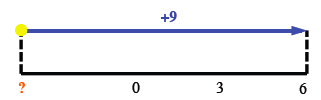### Home > CC1MN > Chapter 3 Unit 3A > Lesson CC1: 3.2.3 > Problem3-119

3-119.

Solve the number puzzles below. 3-119 HW eTool (CPM)   Homework Help ✎

1. If I add $9$ to my number, I get $6$. What is my number?Based on the number line, what was the original number?

2. If I start at $-5$ on a number line and end up at $-8$, what direction did I move? How many units did I move?

Experiment with the number line on the eTool below and use your observations to answer the question.

3. If I moved up $8$ and then moved down $8$, what can you tell me about my ending position?

• Try making your own number line (this one should be vertical).
Draw arrows $8$ up and then $8$ down and see where you land.

It is the same as the starting position. Can you see why?